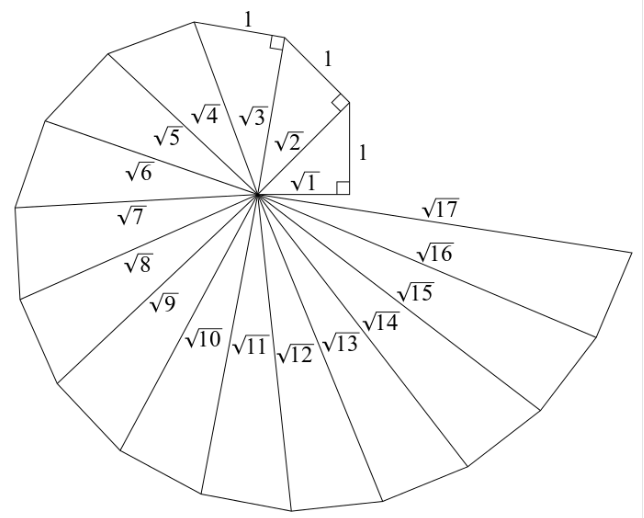Newbie

# Classroom activity (Constructing the ‘square root spiral’) : Take a large sheet of paper and construct the ‘square root spiral’ in the following fashion. Start with a point O and draw a line segment OP1 of unit length. Draw a line segment P1P2 perpendicular to OP1 of unit length (see Fig. 1.9). Now draw a line segment P2P3 perpendicular to OP2. Then draw a line segment P3P4 perpendicular to OP3. Continuing in Fig. 1.9 :… Q.4

• 1

Today i am solving the ncert class 9th solution of chapter number systems  What is the best solution of exercise 1.2 question number 4 , how is solve this important question of this chapter give me the simplest form to solve this question.Classroom activity (Constructing the ‘square root spiral’) : Take a large sheet of paper and construct the ‘square root spiral’ in the following fashion. Start with a point O and draw a line segment OP1 of unit length. Draw a line segment P1P2 perpendicular to OP1 of unit length (see Fig. 1.9). Now draw a line segment P2P3 perpendicular to OP2. Then draw a line segment P3P4 perpendicular to OP3. Continuing in Fig. 1.9Constructing this manner, you can get the line segment Pn-1Pn by square root spiral drawing a line segment of unit length perpendicular to OPn-1. In this manner, you will have created the points P2, P3,….,Pn,… ., and joined them to create a beautiful spiral depicting √2, √3, √4, …

Share

1. n:Step 1: Mark a point O on the paper. Here, O will be the center of the square root spiral.

Step 2: From O, draw a straight line, OA, of 1cm horizontally.

Step 3: From A, draw a perpendicular line, AB, of 1 cm.

Step 4: Join OB. Here, OB will be of √2

Step 5: Now, from B, draw a perpendicular line of 1 cm and mark the end point C.

Step 6: Join OC. Here, OC will be of √3

Step 7: Repeat the steps to draw √4, √5, √6….

• 0